Name ______________________ # ____ Plotting Data Scaling an axis Step 1 - Largest data/(number of divisions)  ex) 32 sec/20 boxes = 1.6 Step 2 - Round up: 1.6 becomes 2
 1. Base your answers to questions a through d on the information, diagram, and data table below. The diagram shows a light string attached to mass m forming a pendulum of length . One complete vibration of the pendulum consists of mass m moving from position A to position B and back to position A. The data table shows the results of an experiment measuring the time for 10 complete vibrations of the pendulum for various pendulum lengths.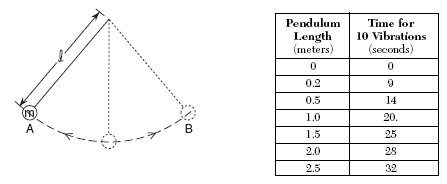Using the information in the data table, construct a graph on the grid provided on the right, following the directions below.   a) Mark an appropriate scale on the axis labeled “Time for 10 Vibrations.” b) Plot the data points for time for 10 vibrations versus length. c) Draw the best-fit curve. d) Determine the period of the 1.0-meter pendulum.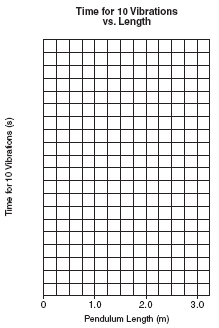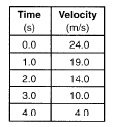2. A car is traveling due north at 24.0 meters per second when the driver sees an obstruction on the highway. The data table below shows the velocity of the car at 1.0 second intervals as it is brought to rest on the straight, level highway. Using the information in the data table, construct a graph on the grid provided on your answer paper, following the directions below. The grid below is provided for practice purposes only. Be sure your final answer appears on your answer paper.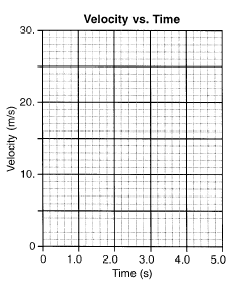a) Plot the data points for velocity versus time. [I] b) Draw the best-fit line. c)  Using your graph, determine the acceleration of the car. [Show all calculations, including the equation and substitution with units.]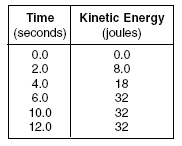The table lists the kinetic energy of a 4.0-kilogram mass as it travels in a straight line for 12.0 seconds. 3.  Directions: Using the information in the data table, construct a graph on the grid provided below, following the directions below. a) Mark an appropriate scale on the axis labeled "Kinetic Energy (J).b) Plot the data points for kinetic energy versus time. c) Calculate the speed of the mass at 10.0 seconds. [Show all work, including the equation and substitution with units.]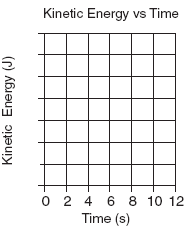d) Compare the speed of the mass at 6.0 seconds to the speed of the mass at 10.0 seconds.      e) Using dimensional analysis, show that the expression v2 /d has the same units as acceleration. [Show all the steps used to arrive at your answer.]4.  Using the information in the data table, construct a line graph on the grid provided in your answer booklet, following the directions below.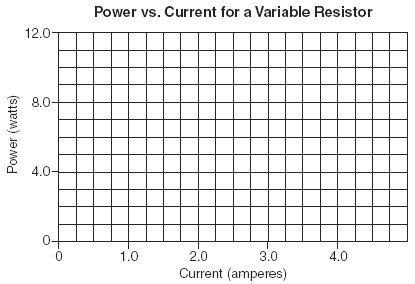e) What is the physical significance of the slope of the graph? ___________ a) Plot the data points for power versus current. b) Draw the best-fit line. c) Using your graph, determine the power delivered to the circuit at a current of 3.5 amperes. ___________ d) Calculate the slope of the graph. [Show all calculations, including the equation and substitution with units.]

 5.  Base your answers to questions a through d on the information and data table below. In an experiment, a student measured the length and period of a simple pendulum. The data table lists the length (ℓ) of the pendulum in meters and the square of the period (T2 ) of the pendulum in seconds2 . Directions (a – d): Using the information in the data table, construct a graph on the grid provided in your answer booklet, following the directions below. a) Plot the data points for the square of period versus length. b)  Draw the best-fit straight line.  c)  Using your graph, determine the time in seconds it would take this pendulum to make one complete swing if it were 0.200 meter long.   The period of a pendulum is related to its length by the formula:where g represents the acceleration due to gravity. d)  Explain how the graph you have drawn could be used to calculate the value of g. [You do not need to perform any actual calculations.]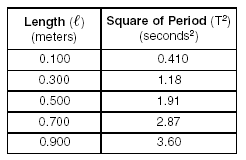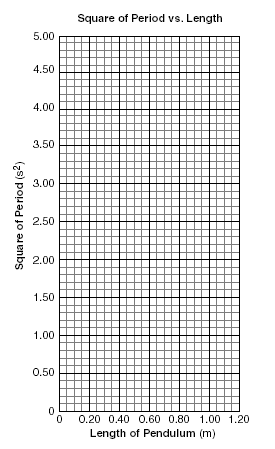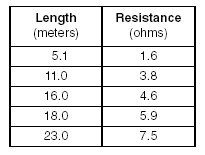6. Base your answers to questions a through d on the information and data table below. An experiment was performed using various lengths of a conductor of uniform cross-sectional area. The resistance of each length was measured and the data recorded in the table below. Directions (a – c): Using the information in the data table, construct a graph on the grid provided in your answer booklet, following the directions below.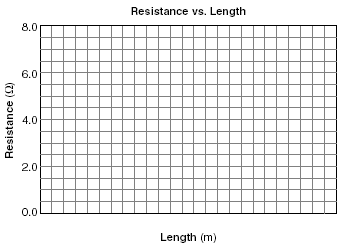a. Mark an appropriate scale on the axis labeled "Length (m)."  b. Plot the data points for resistance versus length. c. Draw the best-fit line. d. Calculate the slope of the best-fit line. [Show all work, including the equation and substitution with units.]